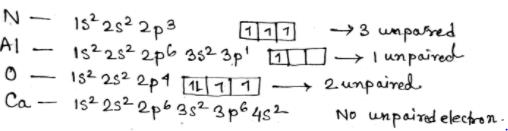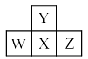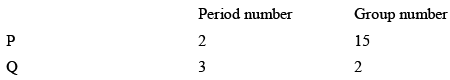Courses

# Test: Periodic Properties- 3

## 30 Questions MCQ Test Inorganic Chemistry | Test: Periodic Properties- 3

Description
This mock test of Test: Periodic Properties- 3 for Chemistry helps you for every Chemistry entrance exam. This contains 30 Multiple Choice Questions for Chemistry Test: Periodic Properties- 3 (mcq) to study with solutions a complete question bank. The solved questions answers in this Test: Periodic Properties- 3 quiz give you a good mix of easy questions and tough questions. Chemistry students definitely take this Test: Periodic Properties- 3 exercise for a better result in the exam. You can find other Test: Periodic Properties- 3 extra questions, long questions & short questions for Chemistry on EduRev as well by searching above.
QUESTION: 1

Solution:
QUESTION: 2

Solution:
QUESTION: 3

### Which one of the following elements shows both positive and negative oxidation states:

Solution:
QUESTION: 4

Which of the following arrangements shows the correct order of decreasing paramagnetism:

Solution:

Explanation :The correct order of decreasing paramagnetism is :

N > O > Al > Ca

QUESTION: 5

Which of the following bond is strongest

Solution:

Because of small differences in electronegativities, the C−H bond is generally regarded as being non-polar. The real reason here is atomic size. The hydrogen atom is much smaller than the carbon atom. Smaller bonds lead to higher bond energy; therefore C−H bond has higher bond enthalpy than the C−C bond.

QUESTION: 6

The elements with the lowest atomic number that has a ground state electronic configuration of (n – 1) d6ns2 is located in the:

Solution:
QUESTION: 7

The atomic numbers of the metallic and non-metallic elements which are liquid at room temperature respectively are:

Solution:
QUESTION: 8

In the periodic table, metallic character of the elements shows one of the following trend:

Solution:
QUESTION: 9

Which of the following represents the correct order of increasing first ionization enthalpy for Ca,Ba,S,Se andAr?

Solution:
QUESTION: 10

Transit ion metals are not characterized by:

Solution:
QUESTION: 11

Sodium generally does not shown oxidation state of +2, because of its:

Solution:
QUESTION: 12

Which of the following pairs of molecules have the almost identical bond dissociation energy:

Solution:
QUESTION: 13

According to modern periodic law the properties of elements repeat at regular intervals when the elements are arranged in order of:

Solution:
QUESTION: 14

Consider the following four elements, which are represented according to long form of periodic table.Here W, Y and Z are left, up and right elements with respect to the element ‘X’ and ‘X’ belongs to 16th group and 3rd period. Then according to given informat ion the incorrect statement regarding given elements is:

Solution:
QUESTION: 15

In the fourth period of the periodic table, how many elements have one or more 4d electrons:

Solution:
QUESTION: 16

Assuming that elements are formed to complete the seventh period, what would be the atomic number of the alkaline earth metal of the eighth period:

Solution:
QUESTION: 17

Which of the following represents an excited state of an atom:

Solution:
QUESTION: 18

Choose the correct statement regarding transition elements:

Solution:
QUESTION: 19

Which one of the following is a different pair:

Solution:
QUESTION: 20

Zn and Cd metals do not show variable valency because:

Solution:
QUESTION: 21

A compound contains three elements A, B and C, if the oxidation number of A = +2, B = +5 and C = –2, the possible formula of the compound is:

Solution:

A3(BC4)2

=3×2+[5+(−2×4)]2=0

.

QUESTION: 22

The period number and group number of “Tantalum” (Z = 73) are respectively:

Solution:
QUESTION: 23

Which of the following pair of elements belongs to the same period:

Solution:

Both Calcium and Zinc belongs to period 4

QUESTION: 24

Consider the following electronic configuration of an element (P):

[Xe] 4f145d16s2

The correct statement about element ‘P’ is:

Solution:
QUESTION: 25

Which of the following metal is highest electropositive (metallic) in nature:

Solution:
QUESTION: 26

Which of the following species must have maximum number of electrons in ‘dxy’ orbital:

Solution:
QUESTION: 27

Which property decreases from left to right across the periodic table and increases from top to bottom:

(II) Electronegativity
(III) Ionization energy
(IV) Metallic character

Solution:
QUESTION: 28

Consider the following information about element P and Q:Then formula of the compound formed by P and Q element is:

Solution:
QUESTION: 29

Which electronic configuration must represent an atom in an excited state:

Solution:
QUESTION: 30

Among NaF , NaCl , NaBr and Nal , the NaF has highest melting point because

Solution: